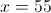# High School Math : Acute / Obtuse Triangles

## Example Questions

### Example Question #5 : Acute / Obtuse Isosceles Triangles

The base angle of an isosceles triangle is 15 less than three times the vertex angle.  What is the vertex angle?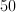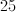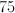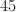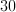Explanation:

Every triangle contains 180 degrees.  An isosceles triangle has one vertex angle and two congruent base angles.

Let= vertex angle and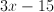= base angle

So the equation to solve becomes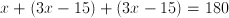.

### Example Question #6 : Acute / Obtuse Isosceles Triangles

The base angle of an isosceles triangle is ten less than twice the vertex angle.  What is the vertex angle?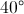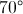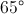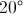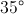Explanation:

Every triangle has 180 degrees.  An isosceles triangle has one vertex angle and two congruent base angles.

Let= vertex angle and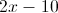= base angle

So the equation to solve becomes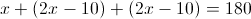So the vertex angle is 40 and the base angles is 70

### Example Question #1 : Isosceles Triangles

The base angle of an isosceles triangle is 10 more than twice the vertex angle.  What is the vertex angle?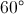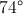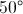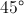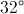Explanation:

Every triangle has 180 degrees.  An isosceles triangle has one vertex angle and two congruent base angles.

Let= the vertex angle and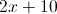= the base angle

So the equation to solve becomes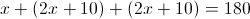The vertex angle is 32 degrees and the base angle is 74 degrees

### Example Question #11 : Isosceles Triangles

In an isosceles triangle, the vertex angle is 15 less than the base angle.  What is the base angle?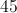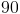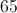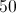Explanation:

Every triangle has 180 degrees.  An isosceles triangle has one vertex angle and two congruent base angles.

Let= base angle and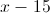= vertex angle

So the equation to solve becomes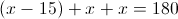Thus, 65 is the base angle and 50 is the vertex angle.

### Example Question #12 : Isosceles Triangles

In an isosceles triangle the vertex angle is half the base angle.  What is the vertex angle?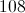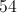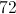Explanation:

Every triangle has 180 degrees.  An isosceles triangle has one vertex angle and two congruent base angles.

Let= base angle and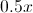= vertex angle

So the equation to solve becomes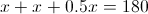, thus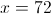is the base angle and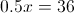is the vertex angle.

### Example Question #11 : How To Find An Angle In An Acute / Obtuse Triangle

If the average of the measures of two angles in a triangle is 75o, what is the measure of the third angle in this triangle?

65°

75°

50°

30°

40°

30°

Explanation:

The sum of the angles in a triangle is 180o:  a + b + c = 180

In this case, the average of a and b is 75:

(a + b)/2 = 75, then multiply both sides by 2

(a + b) = 150, then substitute into first equation

150 + c = 180

c = 30

### Example Question #12 : How To Find An Angle In An Acute / Obtuse Triangle

Which of the following can NOT be the angles of a triangle?

45, 90, 100

30.5, 40.1, 109.4

1, 2, 177

45, 45, 90

30, 60, 90

45, 90, 100

Explanation:

In a triangle, there can only be one obtuse angle. Additionally, all the angle measures must add up to 180.

### Example Question #13 : How To Find An Angle In An Acute / Obtuse Triangle

Let the measures, in degrees, of the three angles of a triangle be x, y, and z. If y = 2z, and z = 0.5x - 30, then what is the measure, in degrees, of the largest angle in the triangle?

96
30
48
60
108
Explanation:

The measures of the three angles are x, y, and z. Because the sum of the measures of the angles in any triangle must be 180 degrees, we know that x + y + z = 180. We can use this equation, along with the other two equations given, to form this system of equations:

x + y + z = 180

y = 2z

z = 0.5x - 30

If we can solve for both y and x in terms of z, then we can substitute these values into the first equation and create an equation with only one variable.

Because we are told already that y = 2z, we alreay have the value of y in terms of z.

We must solve the equation z = 0.5x - 30 for x in terms of z.

z + 30 = 0.5x

Mutliply both sides by 2

2(z + 30) = 2z + 60 = x

x = 2z + 60

Now we have the values of x and y in terms of z. Let's substitute these values for x and y into the equation x + y + z = 180.

(2z + 60) + 2z + z = 180

5z + 60 = 180

5z = 120

z = 24

Because y = 2z, we know that y = 2(24) = 48. We also determined earlier that x = 2z + 60, so x = 2(24) + 60 = 108.

Thus, the measures of the three angles of the triangle are 24, 48, and 108. The question asks for the largest of these measures, which is 108.

### Example Question #14 : How To Find An Angle In An Acute / Obtuse Triangle

Angles x, y, and z make up the interior angles of a scalene triangle. Angle x is three times the size of y and 1/2 the size of z. How big is angle y.

36

108

54

42

18

18

Explanation:

We know that the sum of all the angles is 180. Using the rest of the information given we can write the other two equations:

x + y + z = 180

x = 3y

2x = z

We can solve for y and z in the second and third equations and then plug into the first to solve.

x + (1/3)x + 2x = 180

3[x + (1/3)x + 2x = 180]

3x + x + 6x = 540

10x = 540

x = 54

y = 18

z = 108

### Example Question #1 : How To Find An Angle In An Acute / Obtuse Triangle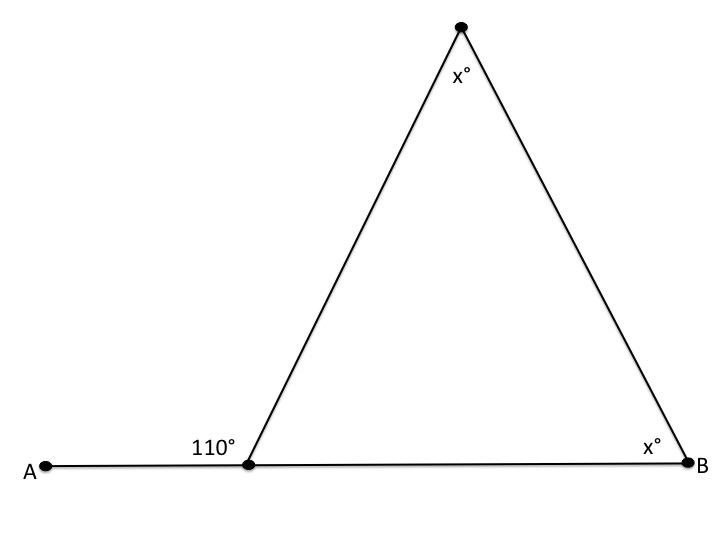In the picture above,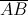is a straight line segment. Find the value of.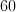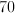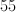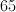Explanation:

A straight line segment has 180 degrees. Therefore, the angle that is not labelled must have: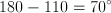We know that the sum of the angles in a triangle is 180 degrees. As a result, we can set up the following algebraic equation: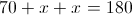Subtract 70 from both sides: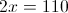Divide by 2: